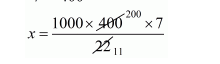# A cubic cm of gold is drawn into a wire 0.1 mm in diameter,Question:

A cubic cm of gold is drawn into a wire 0.1 mm in diameter, find the length of the wire.

Solution:

Let x be the length of wire.

Clearly, the volume of gold = volume of wire.

{Volume of gold = 1 cm3 = 1000 mm}

$1000=\pi\left(\frac{0.1}{2}\right)^{2} \times x$

$\frac{22}{7} \times \frac{1}{400} \times x=1000$$=\frac{1400 \times 1000}{11}$

$=127300 \mathrm{~mm}$

$x=127.3 \mathrm{~m}$

Hence, the length of wire is 127.3 m.